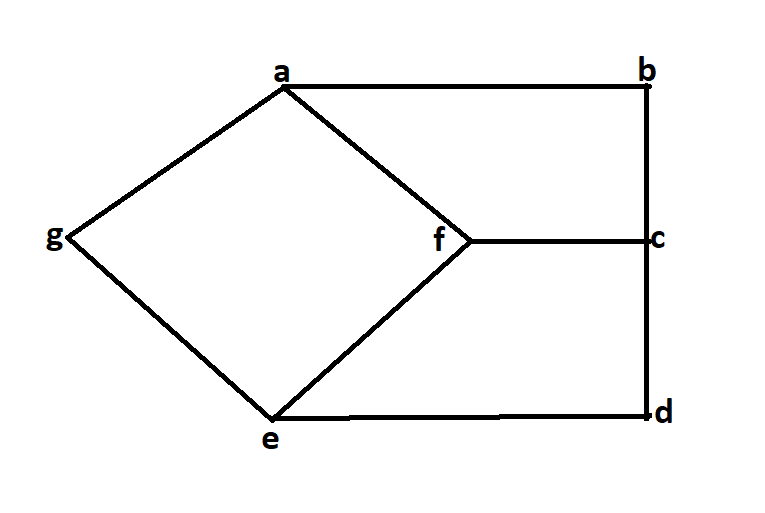# Basic Properties of a Graph

A Graph is a non-linear data structure consisting of nodes and edges. The nodes are sometimes also referred to as vertices and the edges are lines or arcs that connect any two nodes in the graph.

Properties of Graphs are basically used for characterization of graphs depending on their structures. We defined these properties in specific terms that pertain to the domain of graph theory. In this article, we are going to discuss some properties of Graphs these are as follows:

1. Distance between two Vertices
It is basically the number of edges that are available in the shortest path between vertex A and vertex B.If there is more than one edge which is used two connect two vertices then we basically considered the shortest path as the distance between these two vertices.

```Notation used :
d(A, B)
here function d is basically showing the distance between vertex A and vertex B.
```

Let us understand this using an example:In the above diagram, let’s try to find the distance between vertices b and d.

```d(b, c)
We can go from vertex b to vertex d in different ways such as
1.ba, af, fe, ed here the d(b, c) will be 4.
2.ba, af, fc, cd here the d(b, c) will be 4.
3.bc, cf, fe, ed here the d(b, c) will be 4.
4.bc, cd here the d(b, c) will be 2.
hence the minimum distance between vertex b and vertex d is 2.
```
2. Eccentricity of a Vertex
Maximum distance from a vertex to all other vertices is considered as the Eccentricity of that vertex.

```Notation used:
e(V)
here e(v) determines the eccentricity of vertex V.
```

Let us try to understand this using following example.```From the above diagram lets try to find the eccentricity of vertex b.
e(b)
d(b, a)=1
d(b, c)=1
d(b, d)=2
d(b, e)=3
d(b, f)=2
d(b, g)=2
Hence the eccentricity of vertex b is 3
```
3. Radius of a Connected Graph
The minimum value of eccentricity from all vertices is basically considered as the radius of connected graph.

```Notation used:
r(G)
here G is the connected graph.
```

Let us try to understand this using following example.```From the above diagram:
r(G) is 2.
Because the minimum value of eccentricity from all vertices is 2.
```
4. Diameter of A Connected Graph
Unlike the radius of the connected graph here we basically used the maximum value of eccentricity from all vertices to determine the diameter of the graph.

```Notation used:
d(G)
where G is the connected graph.
```

Let us try to understand this using following example.```From the above diagram:
d(G) is 3.
Because the maximum value of eccentricity from all vertices is 3.
```
5. Central Point and Centre
The vertex having minimum eccentricity is considered as the central point of the graph.And the sets of all central point is considered as the centre of Graph.

```if
e(V)=r(G)
then v is the central point.
```

Let us try to understand this using following example.```In the above diagram the central point will be f.
because
e(f)=r(G)=2
hence f is considered as the central point of graph.
Hence f is also the centre of the graph.
```

GeeksforGeeks has prepared a complete interview preparation course with premium videos, theory, practice problems, TA support and many more features. Please refer Placement 100 for details

My Personal Notes arrow_drop_upCheck out this Author's contributed articles.

If you like GeeksforGeeks and would like to contribute, you can also write an article using contribute.geeksforgeeks.org or mail your article to contribute@geeksforgeeks.org. See your article appearing on the GeeksforGeeks main page and help other Geeks.

Please Improve this article if you find anything incorrect by clicking on the "Improve Article" button below.

Article Tags :
Practice Tags :

2

Please write to us at contribute@geeksforgeeks.org to report any issue with the above content.